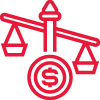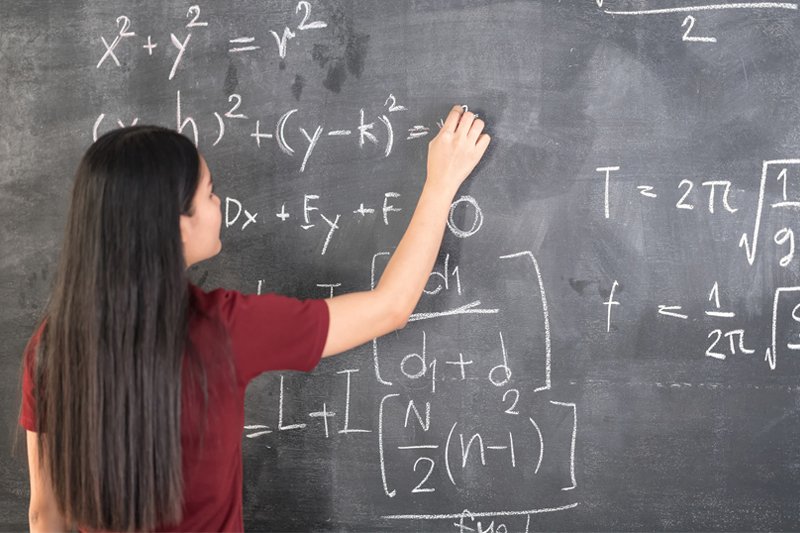# Lower Secondary Math

### Einstein Lower Secondary Math

Lower secondary Math covered in sec 1 and sec 2 are the fundamentals in Math which the students must master and excel in before taking on the E Math and A Math courses in Sec 3 and Sec 4.

In Sec 1 where there are express, normal academic and normal technical streams, the Math subject is tailored to match the students’ learning abilities according to the stream that they are posted to. Real numbers and algebra covered in Sec 1 will largely make up their understanding of the basics of secondary Math.

In particular, Sec 2 is the most crucial year in terms of learning the secondary Math fundamentals. Sec 2 Math covers the bulk of the basics in algebra, namely expansion, factorisation, manipulation, solving quadratic and simultaneous equations. These are the topics that deepens the understanding of secondary school Math and greatly affects the students in solving the questions in Sec 3 and Sec 4 E Math and A Math.

Through the lower secondary Math Achievers’ tuition program, Einstein is committed to piece together the building blocks of Math to develop a strong mathematical mind.

### Program HighlightsStrong Fundamentals
– Formula retention
– Thorough understanding
– Strong sense of exam based questionsStep By Step Mastery
– Clear and systematic
– Apply methods confidently
– Minimise errorsPerformance Driven
– Improve analytical skills
– Raise performance

Prepare for your Mathematics exams with Einstein. Since 2006, we have helped students of different ability levels to score in the school exams. Our Math teaching methodology has proven to be a favourite with our students – super clear, systematic and successful.

### Star Features Of Our S1 & S2 Math Classes##### Small class size

1 to 7 students##### Flat Monthly Fees

4 weeks or 5 weeksNo deposit

No lump sum fees

2 x 1.5h

Weekly

2.5h

Weekly

1.5h

Weekly

2 x 1.5h

Weekly

2.5h

Weekly

1.5h

Weekly

### Mathematics Program Coverage##### Real numbers
• Master the basis of Math to solve different operations involving addition, subtraction, multiplication, division of numbers, decimals and fractions effortlessly.

##### Algebra
• Attain the full understanding of the most important topic in secondary school Math.  Develop effective techniques to solve algebra step by step with clear representation that is easily understood and highly applicable in all kinds of algebra questions such as expansion, factorisation, simplification and solving equations.

##### Graph and constructions
• Pick up the techniques of constructing shapes and bisectors neatly.  Draw curves and straight lines pm graph papers using rulers, french curve or flexible curve.  Mark out the points and present all the working on the graph clearly with a smooth curve or straight line.
##### Mensuration
• Internalise the formulas of cylinfer, cone, sphere, pyramid and apply it onto the question to find out the volume and total surface area correctly.

##### Statistics
• Draw out bar graphs, histograms and pie charts to present the data accurately and answer the questions with full workings and steps shown clearly especially on mean, median, mode and comparison between 2 sets of data.

##### Other topics
• All other topics in MOE Sec1 and Sec2 Math syllabus will be covered comprehensively.